# How to Calculate and Solve for Semi-Log Pressure-Time Slope, Permeability-Thickness Product, Oil Rate, Oil FVF, Oil Viscosity in Well Testing | Nickzom Calculator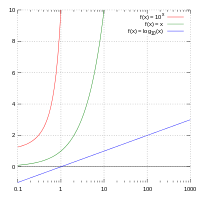The image above represents semi-log pressure-time slope.

To compute for the semi-log pressure-time slope, four essential parameters are needed and these parameters are Permeability-Thickness Product (kh), Oil Rate (Qo), Oil Viscosity (μo) and Oil FVF (Bo).

The formula for calculating the semi-log pressure-time slope:

m = 162.6QoBoμo / kh

Where;

m = Semi-Log Pressure-Time Slope
kh = Permeability-Thickness Product
Qo = Oil Rate
μo = Oil Viscosity
Bo = Oil FVF

Let’s solve an example;
Find the semi-log pressure-time slope, when permeability-thickness product is 12, oil rate is 17, oil viscosity is 21 and oil FVF is 13.7.

This implies that;

kh = Permeability-Thickness Product = 12
Qo = Oil Rate = 17
μo = Oil Viscosity = 21
Bo = Oil FVF = 13.7

m = 162.6QoBoμo / kh
m = 162.6 x 17 x 13.7 x 21 / 12
m = 795260.34 / 12
m = 66271.69

Therefore, the semi-log pressure-time slope is 66271.69.

Calculating the Permeability-Thickness Product when Semi-Log Pressure-Time Slope, Oil Rate, Oil Viscosity and Oil FVF is Given.

kh = 162.6QoBoμo / m

Where;

kh = Permeability-Thickness Product
m = Semi-Log Pressure-Time Slope
Qo = Oil Rate
μo = Oil Viscosity
Bo = Oil FVF

Let’s solve an example;
Find the permeability-thickness product, when semi-log pressure-time slope is 90, oil rate is 23, oil viscosity is 27 and oil FVF is 14.

This implies that;

m = Semi-Log Pressure-Time Slope = 90
Qo = Oil Rate = 23
μo = Oil Viscosity = 27
Bo = Oil FVF = 14

kh = 162.6QoBoμo / m
kh = 162.6 x 23 x 14 x 27 / 90
kh = 1413644.4 / 90
kh = 15707.16

Therefore, the permeability-thickness product is 15707.16.

Calculating the Oil Rate when Semi-Log Pressure-Time Slope, Permeability-Thickness Product, Oil Viscosity and Oil FVF is Given.

Qo = m x kh / 162.6Boo

Where;

Qo = Oil Rate
m = Semi-Log Pressure-Time Slope
kh = Permeability-Thickness Product
μo = Oil Viscosity
Bo = Oil FVF

Let’s solve an example;
Find the oil rate when semi-log pressure-time slope is 110, permeability-thickness product is 42, oil viscosity is 31 and oil FVF is 11.

This implies that;

m = Semi-Log Pressure-Time Slope = 110
kh = Permeability-Thickness Product = 42
μo = Oil Viscosity = 31
Bo = Oil FVF = 11

Qo = m x kh / 162.6Boμo
Qo = 110 x 42 / 162.6 x 11 x 31
Qo = 4620 / 55446.6
Qo = 0.0833

Therefore, the oil rate is 0.0833.

Calculating the Oil Viscosity when Semi-Log Pressure-Time Slope, Permeability-Thickness Product, Oil Rate and Oil FVF is Given.

μo = m x kh / 162.6BoQo

Where;

μo = Oil Viscosity
m = Semi-Log Pressure-Time Slope
kh = Permeability-Thickness Product
Qo = Oil Rate
Bo = Oil FVF

Let’s solve an example;
Find the oil viscosity when semi-log pressure-time slope is 80, permeability-thickness product is 41, oil rate is 50 and oil FVF is 30.

This implies that;

m = Semi-Log Pressure-Time Slope = 80
kh = Permeability-Thickness Product = 41
Qo = Oil Rate = 50
Bo = Oil FVF = 30

μo = m x kh / 162.6BoQo
μo = 80 x 41 / 162.6 x 30 x 50
μo = 3280 / 243900
μo = 0.0134

Therefore, the oil viscosity is 0.0134.

Calculating the Oil FVF when Semi-Log Pressure-Time Slope, Permeability-Thickness Product, Oil Rate and Oil Viscosity is Given.

Bo = m x kh / 162.6μoQo

Where;

Bo = Oil FVF
m = Semi-Log Pressure-Time Slope
kh = Permeability-Thickness Product
Qo = Oil Rate
μo = Oil Viscosity

Let’s solve an example;
Find the oil FVF when semi-log pressure-time slope is 40, permeability-thickness product is 26, oil rate is 12 and oil viscosity is 10.

This implies that;

m = Semi-Log Pressure-Time Slope = 40
kh = Permeability-Thickness Product = 26
Qo = Oil Rate = 12
μo = Oil Viscosity = 10

Bo = m x kh / 162.6μoQo
Bo = 40 x 26 / 162.6 x 10 x 12
Bo = 1040 / 19512
Bo = 0.0533

Therefore, the oil FVF is 0.0533.

Nickzom Calculator – The Calculator Encyclopedia is capable of calculating the semi-log pressure-time slope.

To get the answer and workings of the semi-log pressure-time slope using the Nickzom Calculator – The Calculator Encyclopedia. First, you need to obtain the app.

You can get this app via any of these means:

To get access to the professional version via web, you need to register and subscribe for NGN 1,500 per annum to have utter access to all functionalities.
You can also try the demo version via https://www.nickzom.org/calculator

Apple (Paid) – https://itunes.apple.com/us/app/nickzom-calculator/id1331162702?mt=8
Once, you have obtained the calculator encyclopedia app, proceed to the Calculator Map, then click on Petroleum under EngineeringNow, Click on Well Testing under Petroleum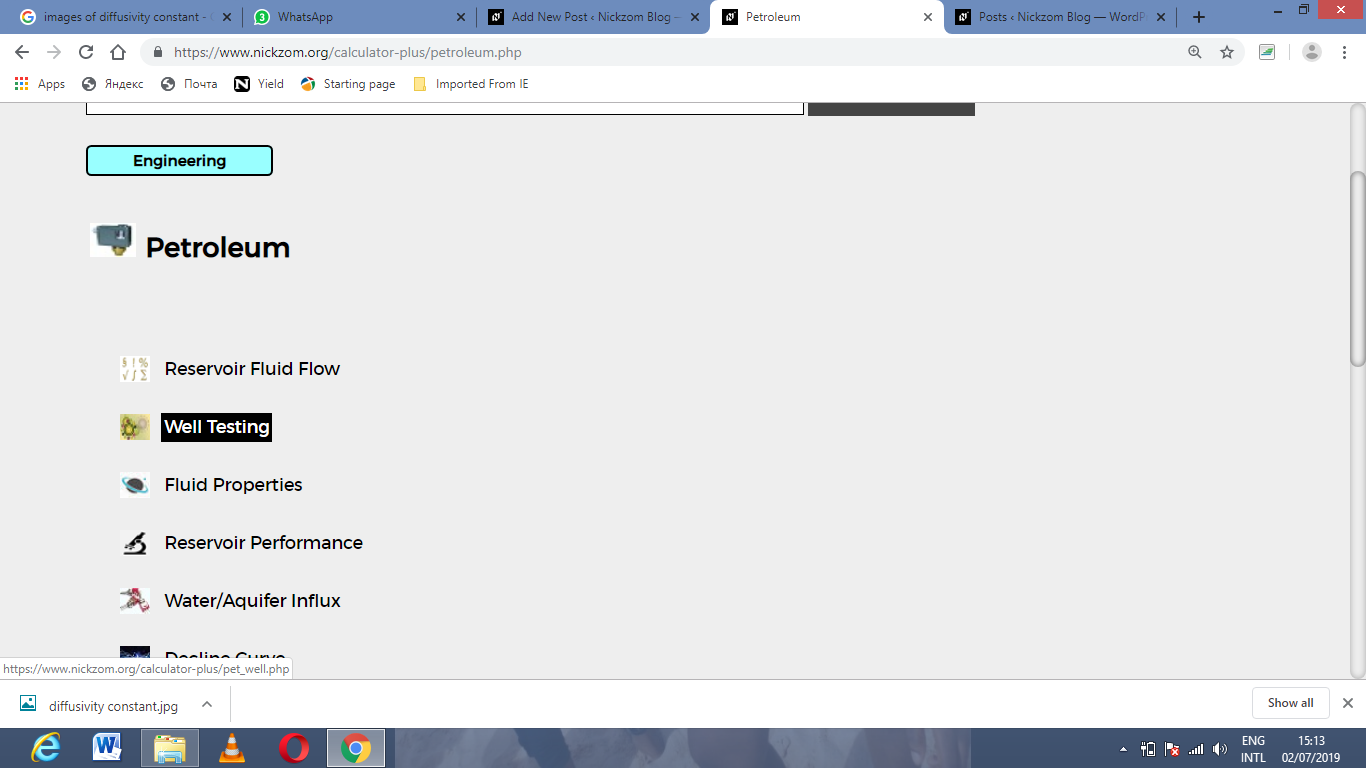Now, Click on Semi-Log Pressure-Time Slope under Well Testing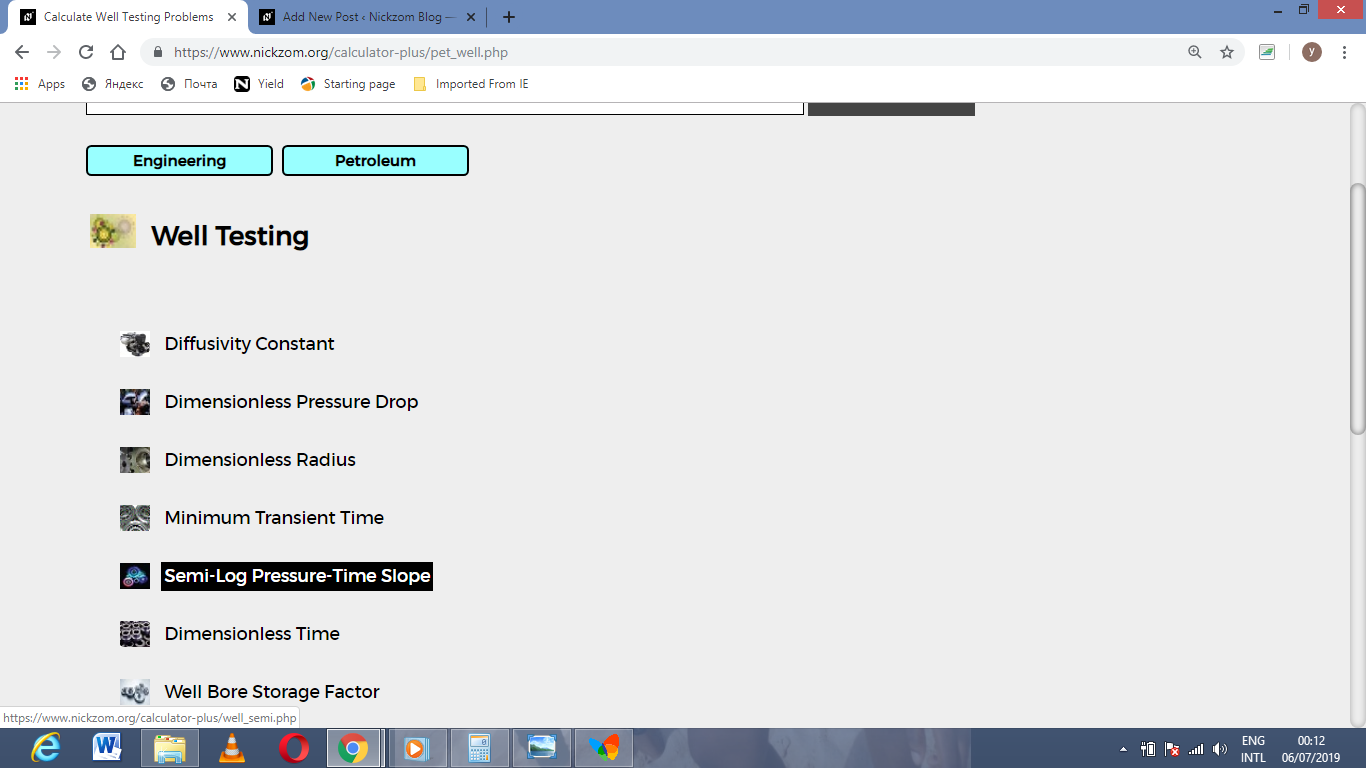The screenshot below displays the page or activity to enter your values, to get the answer for the semi-log pressure-time slope according to the respective parameters which are the Permeability-Thickness Product (kh), Oil Rate (Qo), Oil Viscosity (μo) and Oil FVF (Bo).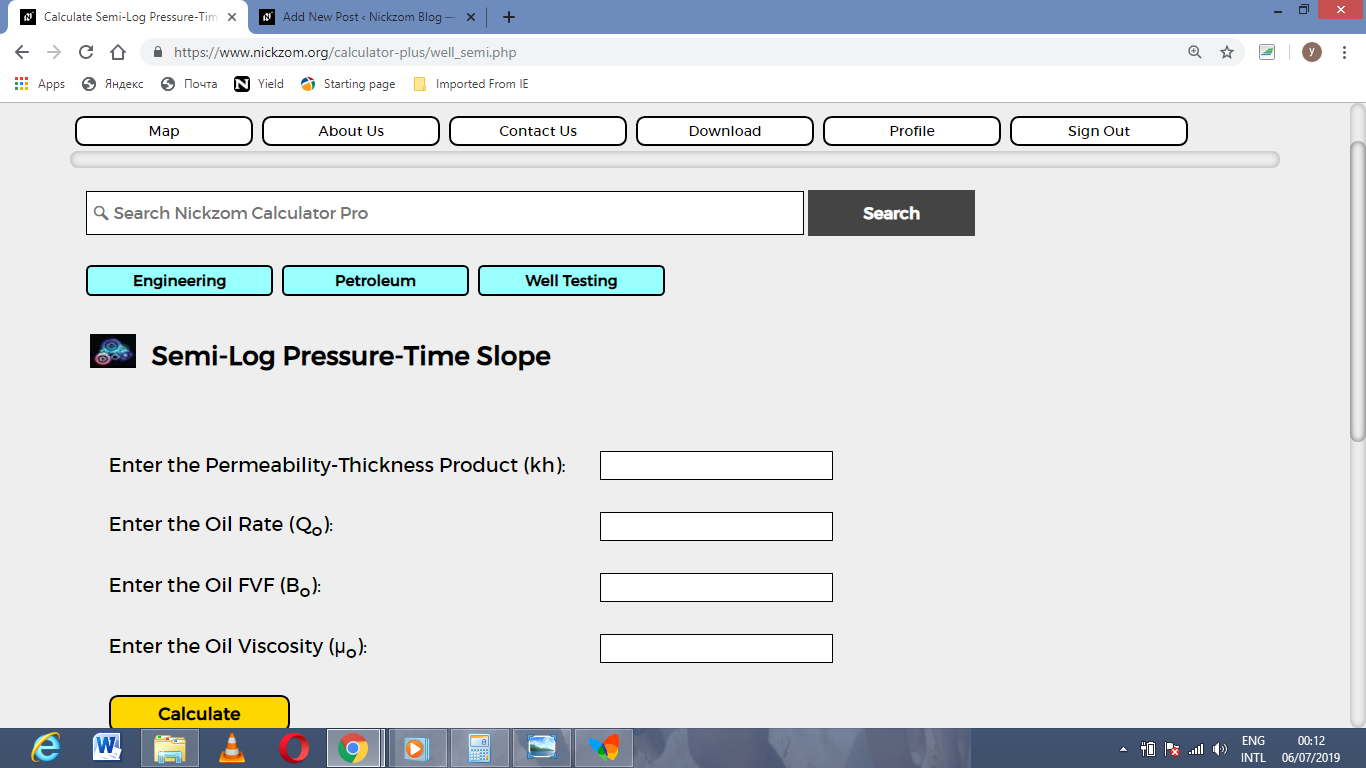Now, enter the values appropriately and accordingly for the parameters as required by the Permeability-Thickness Product (kh) is 12, Oil Rate (Qo) is 17, Oil Viscosity (μo) is 13.7 and Oil FVF (Bo) is 21.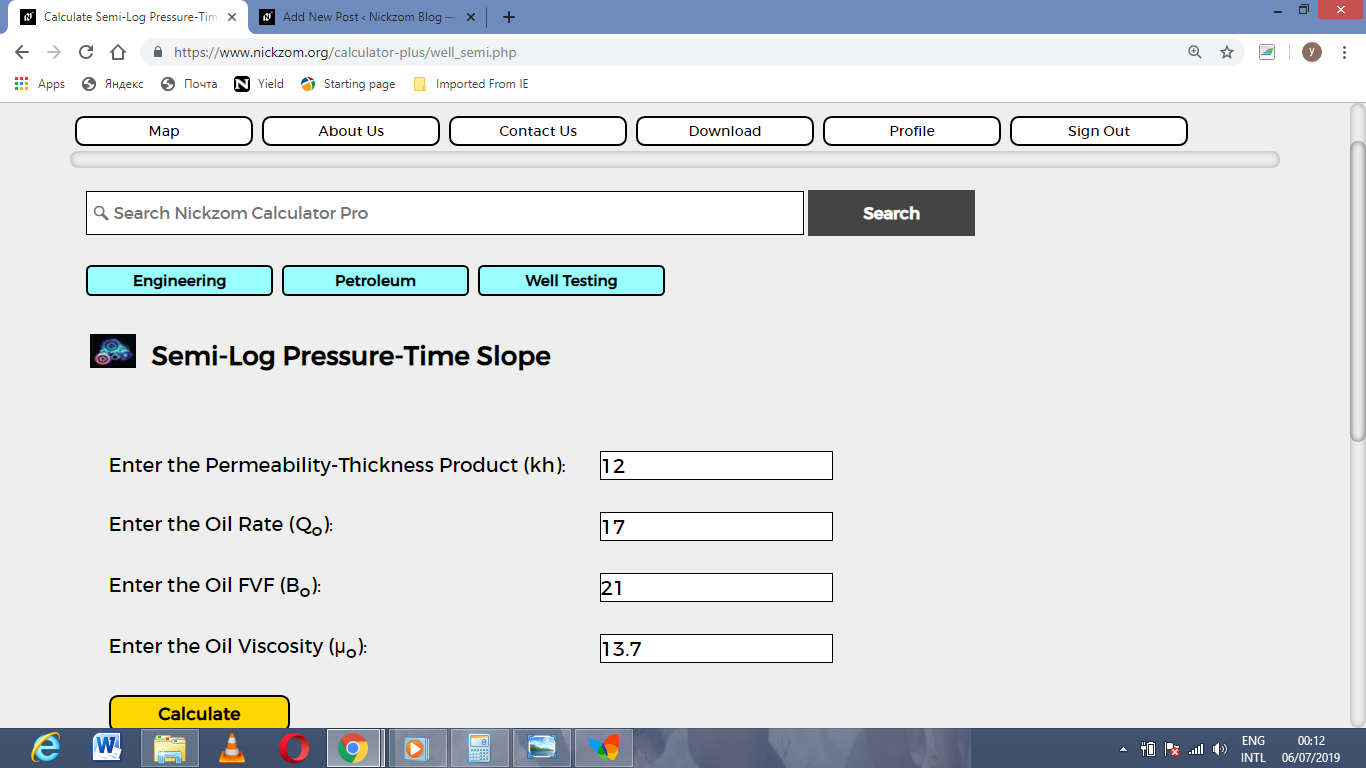Finally, Click on Calculate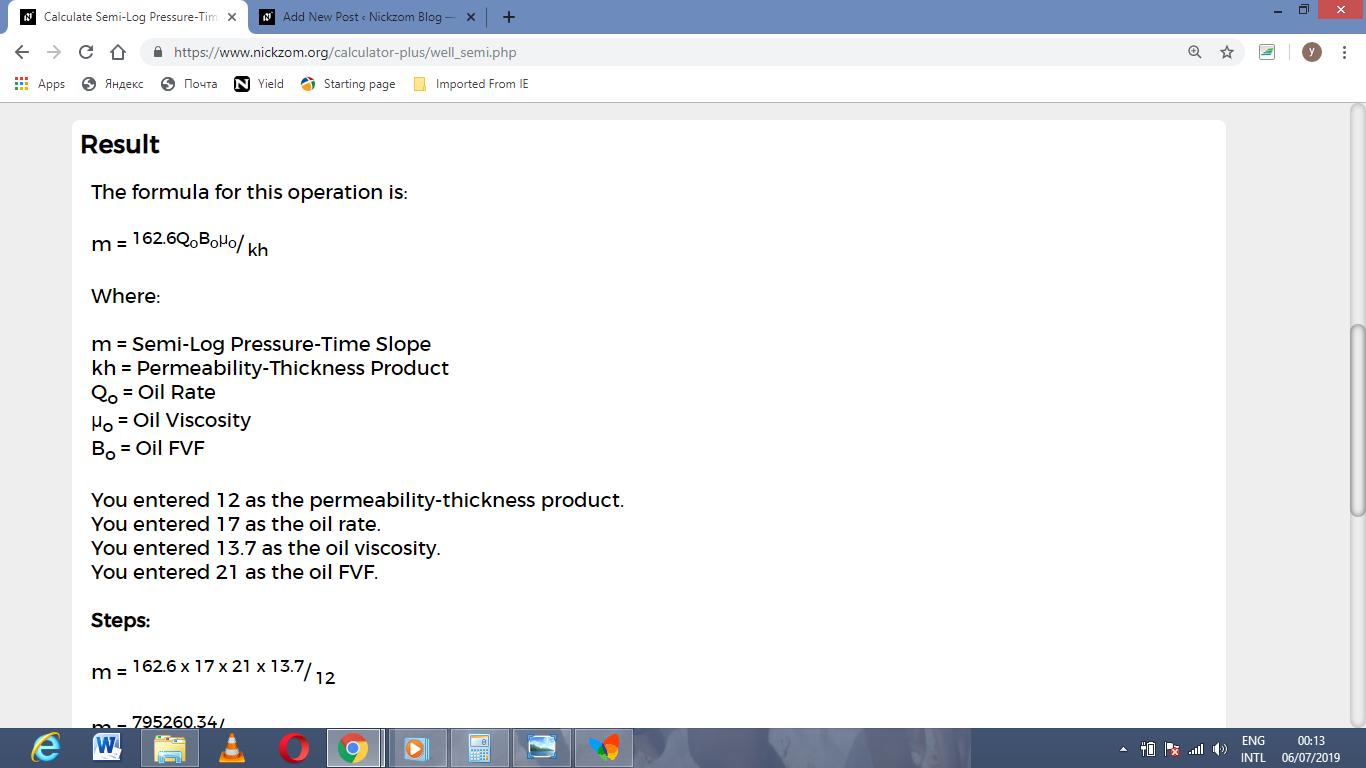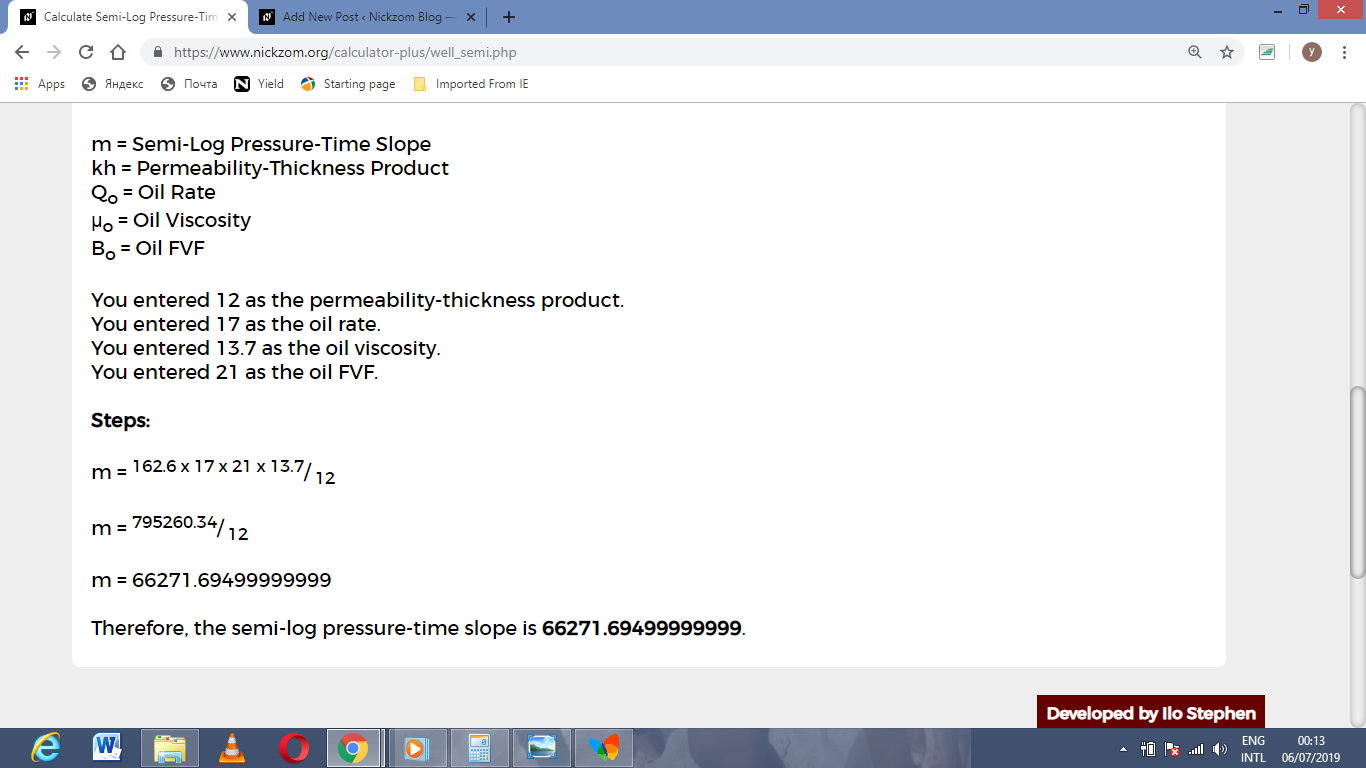As you can see from the screenshot above, Nickzom Calculator– The Calculator Encyclopedia solves for the semi-log pressure-time slope and presents the formula, workings and steps too.